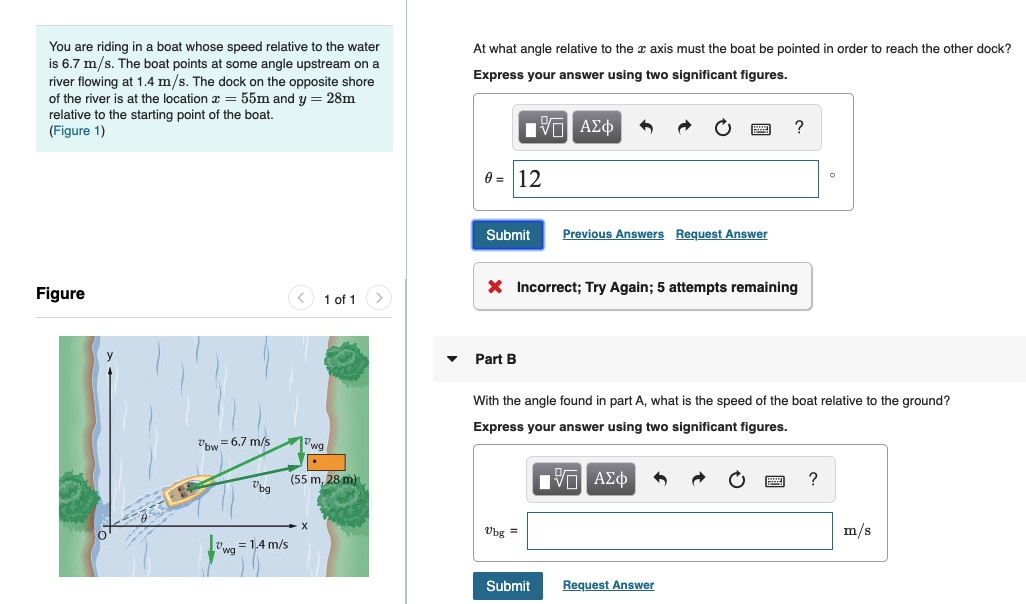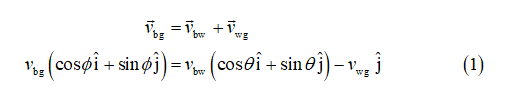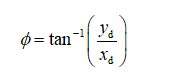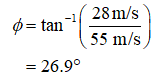# You are riding in a boat whose speed relative to the wateris 6.7 m/s. The boat points at some angle upstream on ariver flowing at 1.4 m/s. The dock on the opposite shoreof the river is at the location r = 55m and y = 28mrelative to the starting point of the boat.(Figure 1)At what angle relative to the r axis must the boat be pointed in order to reach the other dock?Express your answer using two significant figures.Hν ΑΣφ0 = 12Previous AnswersRequest AnswerSubmitX Incorrect; Try Again; 5 attempts remainingFigure< 1 of 1Part BWith the angle found in part A, what is the speed of the boat relative to the ground?Express your answer using two significant figures.wg"bw = 6.7 m/sνα ΑΣφ(55 m, 28 m)m/sUbg == 1,4 m/sRequest AnswerSubmit

Question
13 viewshelp_outlineImage TranscriptioncloseYou are riding in a boat whose speed relative to the water is 6.7 m/s. The boat points at some angle upstream on a river flowing at 1.4 m/s. The dock on the opposite shore of the river is at the location r = 55m and y = 28m relative to the starting point of the boat. (Figure 1) At what angle relative to the r axis must the boat be pointed in order to reach the other dock? Express your answer using two significant figures. Hν ΑΣφ 0 = 12 Previous Answers Request Answer Submit X Incorrect; Try Again; 5 attempts remaining Figure < 1 of 1 Part B With the angle found in part A, what is the speed of the boat relative to the ground? Express your answer using two significant figures. wg "bw = 6.7 m/s να ΑΣφ (55 m, 28 m) m/s Ubg = = 1,4 m/s Request Answer Submit fullscreen
check_circle

Vector equation

From the given vector diagram, write the expression for velocity of the boat with respect to groundThe direction of the velocity of the boat with respect to the groundSubstitution...

### Want to see the full answer?

See Solution

#### Want to see this answer and more?

Solutions are written by subject experts who are available 24/7. Questions are typically answered within 1 hour.*

See Solution
*Response times may vary by subject and question.
Tagged in